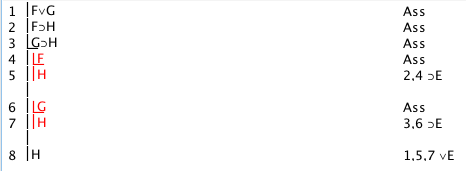# The Proof Panel

## The Proof Panel

##### 5/26/15

The Proof Panel is used for doing proofs or derivations.

#### Selecting items and using Rules

The basic technique is to select as many lines as the Rule requires and then click the Rule (keeping in mind that most computers have a convention for selecting two or more items--the Macintosh convention for selecting more than one item is to click on the first, then press the command key (with the clover-leaf on it) and click on the second, on the third...)

Some Rules of Inference require you to make a choice. For example, ∧I with A and B permits A∧B or B∧A, and you have to choose which you want. You will be asked for a choice.

Some Rules of Inference require you to supply a new formula. For example, ∨I with A permits (A∨<newformula> ) for any well formed formula <newformula>. Supplying a new formula is similar to making a choice, but you have to enter the new formula. Of course, your addition has to be a proper formula. If it is gobbledegook, you will be told so. Usually you have either to supply a proper formula, or Cancel. Entering new formulas is mildly awkward because you have to use the proper symbols, which do not appear on the keyboard. There are several techniques. While doing a proof, clicking on a line will load the formula of that line into an entry panel-- which you can then edit to get what you want. You can copy and paste (or drag and drop) from the symbol palette (and any text that has the proper symbols).

You will notice that much of the time when the program writes messages (for example, error messages) to you those messages will be selected-- this means that you merely have to type anything for the (now unwanted) message to disappear.

Some Rules relate to sub-proofs. Rules that involve only one sub-proof are invoked by selecting the last line of an open but continuing sub-proof; the rule then closes the subproof and writes in the next line. For example, a proof of F⊃G ∴ (F∧F)⊃G starts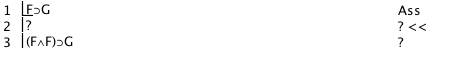Then Ass is used to add the <if-formula>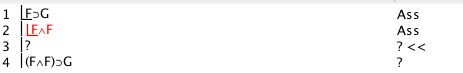The intermediate lines are added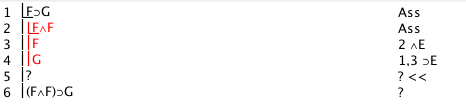At this stage, line 4 is selected (ie the last line of the subproof) and ⊃E clicked, and the rule does the rest.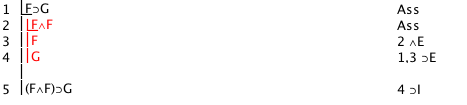Some Rules involve more than one sub-proof. A sub-proof is selected by selecting its last line. A sub-proof has to be ended before it can be selected (otherwise you are simply selecting the last line of a continuing sub-proof).   While a sub-proof is open its lines are available for selection for the rules; but after it has been ended its lines are usually no longer available (as they usually have been proved under assumptions different to those of the proof as a whole). Only the last line of a sub-proof will be selectable and that selection picks the sub-proof not the line. Sub-proofs are usually ended by the Rules that use them, but a sub-proof can be ended simpliciter by invoking the Rule that introduces new assumptions. If the circumstances are right, the Rule will ask you if you want to drop the last new assumption-- dropping the last new assumption closes the previous sub-proof. For example, a derivation of F∨G,F⊃H,G⊃H∴ H starts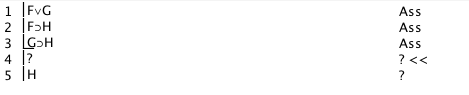Then Ass is used to introduce F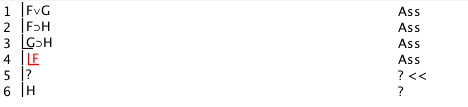Some intermediate lines are added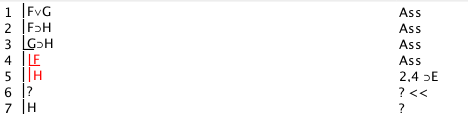Then Ass is used to introduce G. At this point the rule Ass will ask whether the User wants to keep or drop the last assumption. And the last assumption, ie F is dropped to give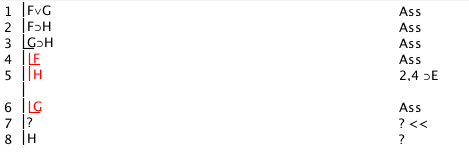Intermediate lines are added.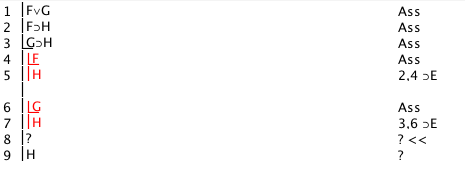Then an Or formula, line 1, a sub-proof, line 5, and the last line of an open sub-proof, line 7 are all selected and ∨E completes the proof.